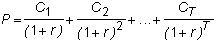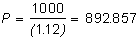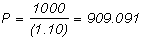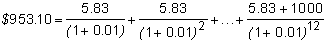﻿ 3.2 Yield to Maturityoffice (412) 967-9367
fax (412) 967-5958
toll-free 1 (800) 214-3480

3.2 Yield to Maturity

 I

n any time value of money problem, there are four essential pieces of information.

1. the magnitude of cash flows over time,

2. the times at which cash flows occur,

3. the interest rate at each time, and

4. the present value or price.

If you have any three of these four pieces of information, you can solve for the fourth piece. In the computations so far, the present value (i.e., price) of a fixed-income security has been calculated using the first three components (the magnitude and timing of cash flows and the interest rate).

If you know the first two and the fourth (the magnitude, timing, and price) variables in the above list, you can calculate what is called the yield to maturity: The yield to maturity is the interest rate at which the price of a bond equals the present value of the cash flows from the bond.

The yield to maturity (sometimes called the internal rate of return from the bond) is a measure of the "average" return from buying a bond. Its calculation requires us to apply the valuation principles developed so far "in reverse." That is, instead of solving for P from some set of spot interest rates that can be different for each period, we solve instead for the interest rate that equates the future cash flows to the current price, P. This interest rate is the yield to maturity.

To help make this concept less abstract, consider a debt contract that pays cash Ct each period for T periods. If the current market value (or price) of this contract is P, then the yield to maturity of the contract is the interest rate, r, that solvesCalculating the yield requires the use of an iterative numerical procedure. Such a procedure is built into the software in Bond Tutor.

Example: Yield to Maturity for a Zero Coupon Bond

Consider a zero-coupon bond with a maturity 12 months from now and a face value of \$1,000. The present value or price of the bond is \$892.857. What is this bond’s yield to maturity?

You can verify that the yield to maturity is 12%:If the price of the bond increases to \$909.09, what is the new yield to maturity? You can check that the new yield is 10%:The new yield to maturity has fallen to 10%. In other words, a rise in the value of the bond is equivalent to a fall in the yield to maturity.

Example: Yield to Maturity for a Coupon Bond

Consider a coupon bond with face value of \$1,000, and a coupon rate of 7% per year, paid monthly, that matures in 12 months. In this case, the bondholder receives \$5.83 every month for 12 months and \$1,000 at the end of 12 months.

If the price or present value of this bond is \$953.1, what is its implied yield to maturity?

You can verify that the implied yield is 1% per month or 12% per year compounded monthly.You may wonder what the difference is between the interest rate used to calculate the value of a bond (the spot interest rate) and the yield to maturity. There is an important distinction between spot interest rates and yields to maturity.

The spot interest rate is the yield to maturity for a zero-coupon bond. That is, it is computed from the market price of a zero-coupon bond. As a result, only the cash flows that occur at the time of maturity are used to determine the spot interest rate. If investors in the market can borrow or lend at the spot rate for any maturity, then the spot rates determine the arbitrage-free price of any fixed-income security.

The yield to maturity is computed given the price of any fixed-income security. It is the interest rate that equates the present value of the future cash inflows to the market price of the security.

In the next topic, Spot Rates and the Yield to Maturity, we contrast these two concepts.